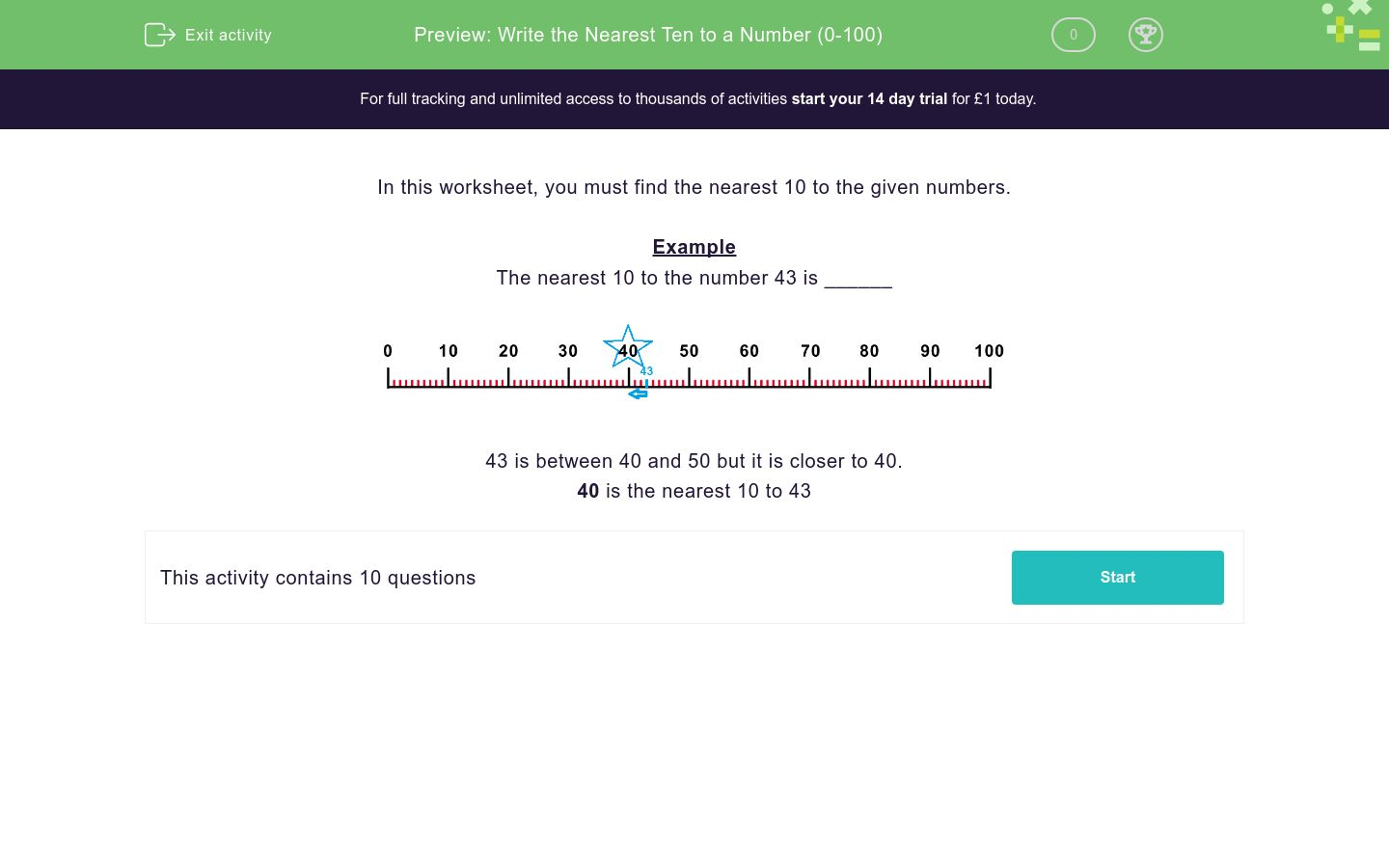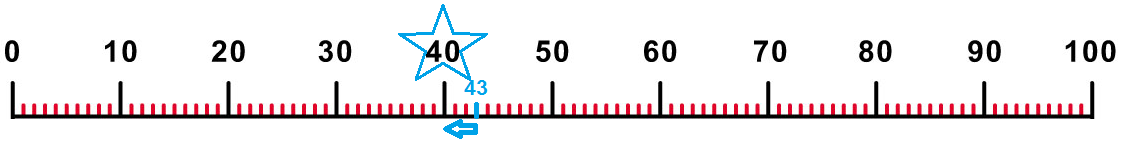# Write the Nearest Ten to a Number (0-100)

In this worksheet, students find the nearest ten to a given number using a number line.Key stage:  KS 1

Curriculum topic:   Number: Number and Place Value

Curriculum subtopic:   Represent Numbers to 100

Difficulty level:### QUESTION 1 of 10

In this worksheet, you must find the nearest 10 to the given numbers.

Example

The nearest 10 to the number 43 is ______43 is between 40 and 50 but it is closer to 40.

40 is the nearest 10 to 43

The nearest 10 to the number 11 is ______.The nearest 10 to the number 83 is ______.The nearest 10 to the number 41 is ______.The nearest 10 to the number 49 is ______.The nearest 10 to the number 89 is ______.The nearest 10 to the number 58 is ______.The nearest 10 to the number 26 is ______.The nearest 10 to the number 53 is ______.The nearest 10 to the number 71 is ______.The nearest 10 to the number 77 is ______.• Question 1

The nearest 10 to the number 11 is ______.10
• Question 2

The nearest 10 to the number 83 is ______.80
• Question 3

The nearest 10 to the number 41 is ______.40
• Question 4

The nearest 10 to the number 49 is ______.50
• Question 5

The nearest 10 to the number 89 is ______.90
• Question 6

The nearest 10 to the number 58 is ______.60
• Question 7

The nearest 10 to the number 26 is ______.30
• Question 8

The nearest 10 to the number 53 is ______.50
• Question 9

The nearest 10 to the number 71 is ______.70
• Question 10

The nearest 10 to the number 77 is ______.80
---- OR ----

Sign up for a £1 trial so you can track and measure your child's progress on this activity.

### What is EdPlace?

We're your National Curriculum aligned online education content provider helping each child succeed in English, maths and science from year 1 to GCSE. With an EdPlace account you’ll be able to track and measure progress, helping each child achieve their best. We build confidence and attainment by personalising each child’s learning at a level that suits them.

Get started